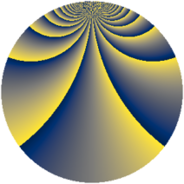# Properties

 Label 912.2.cqLevel $912$ Weight $2$ Character orbit 912.cq Rep. character $\chi_{912}(61,\cdot)$ Character field $\Q(\zeta_{36})$ Dimension $960$ Newform subspaces $1$ Sturm bound $320$ Trace bound $0$

# Related objects

## Defining parameters

 Level: $$N$$ $$=$$ $$912 = 2^{4} \cdot 3 \cdot 19$$ Weight: $$k$$ $$=$$ $$2$$ Character orbit: $$[\chi]$$ $$=$$ 912.cq (of order $$36$$ and degree $$12$$) Character conductor: $$\operatorname{cond}(\chi)$$ $$=$$ $$304$$ Character field: $$\Q(\zeta_{36})$$ Newform subspaces: $$1$$ Sturm bound: $$320$$ Trace bound: $$0$$

## Dimensions

The following table gives the dimensions of various subspaces of $$M_{2}(912, [\chi])$$.

Total New Old
Modular forms 1968 960 1008
Cusp forms 1872 960 912
Eisenstein series 96 0 96

## Trace form

 $$960q + O(q^{10})$$ $$960q + 24q^{10} + 12q^{16} - 144q^{31} + 60q^{32} + 120q^{34} + 12q^{36} - 228q^{38} - 60q^{40} - 60q^{46} + 480q^{49} + 36q^{50} - 24q^{51} - 48q^{52} - 12q^{54} - 72q^{68} + 48q^{69} - 72q^{70} + 48q^{76} + 72q^{78} - 120q^{80} - 120q^{82} - 96q^{85} - 168q^{94} - 84q^{98} + O(q^{100})$$

## Decomposition of $$S_{2}^{\mathrm{new}}(912, [\chi])$$ into newform subspaces

Label Dim. $$A$$ Field CM Traces $q$-expansion
$$a_2$$ $$a_3$$ $$a_5$$ $$a_7$$
912.2.cq.a $$960$$ $$7.282$$ None $$0$$ $$0$$ $$0$$ $$0$$

## Decomposition of $$S_{2}^{\mathrm{old}}(912, [\chi])$$ into lower level spaces

$$S_{2}^{\mathrm{old}}(912, [\chi]) \cong$$ $$S_{2}^{\mathrm{new}}(304, [\chi])$$$$^{\oplus 2}$$